Last updated on 3/6/20

## Collect data with arraysJenny is very much impressed by your work; the results of your calculations have made it possible for her to plan out her idea.

This has inspired her to use this opportunity to see how else she can take advantage of digital modeling to develop her business strategy further.

Now she would like to figure out what part of her business brings her the most profit.  This way she can make sure to give it all the support necessary, while other ventures serve as fun experiments.

In her cafe, she offers 3 categories of items for sale:

• Coffee - couldn't do without it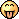• Desserts - an eternal partner of coffee; it goes without saying!

• Gourmet food - for those who'd love to stay for a meal!

They sell in different quantities daily, have different profit margins, different cost of ingredients, and require various amounts of staff time to prepare and serve them.

Jenny wonders if you can help?!

Of course you can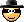!

To solve this problem we will need to learn a few new programming tricks. Specifically, new data types: collections.

A collection is what we call a collection of elements. And the whole collection of elements is associated with one variable.

There 3 types of collections:

• Array - a numerically ordered list

• Dictionary - a list organized by key

• Set - a set of unordered unique values

In this chapter we are going to learn the Array data type.

We are getting into more sophisticated aspects of programming now, so take your time to comprehend the new knowledge.

An array is a numerically ordered list of elements. Which means that each element is associated with a number. That number is called an index. Indexing in Swift starts with 0 (not 1). So the first element is associated with an index 0, second - 1 etc.

Here's an example of an indexed list of elements:

To imagine an array, think of a bookshelf, the first shelf has index 0, the second shelf has index 1, and so on. The last index in an array equals the number of elements in the array - 1. In our example we have 10 elements associated with indices from 0 to 9.

An array may contain any number of elements, including ZERO (an empty array).

#### Array syntax

To declare an array we use square brackets  `[]`   and list all elements inside separated by a comma:

``````[element1, element2, element3]
``````

If there are no elements, then we use empty brackets:

``````[]
``````

What type of elements can we put in an array?

An array elements can be of ANY type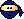.

We already know numeric types, we can arrange integers (  `Int`  ) in an array, for example. If we are creating a calculator app, we would need to store numbers associated with 10 buttons. An array variable could be used to store those numbers:

``````[0, 1, 2, 3, 4, 5, 6, 7, 8, 9]
``````

Strings (  `String`  ) would be another example. If we want to make our calculator color theme customizable, we could store colors in an array: 'yellow', ‘green’ or ‘blue’ etc.

``````["yellow", "green", "blue"]
``````

You can observe the indexing of an array variable in Playground by clicking an eye icon:

Just like for simple variable types, the same rules apply to arrays; it always has a type and it cannot change its type.

All the elements in an array must be of the same type:

``````["yellow", "green", "blue"] // Ok
["yellow", "green", "blue", 12] // Error!
``````

If we don’t yet have any data to put in the array at the time of declaring it, like any other variable we can declare it by specifying the type we intend to use in the future. And for arrays we need to put the type of elements in square brackets  `[String]`   if we intend to use strings.

#### Declaring arrays

The keyword  `Array`  describes an array variable. However, unlike other data types, it's not necessary to use it for the declaration:

``````var myArrayOfStrings: [String] // declaration by specifying the type of elements
var myArrayOfStrings: Array<String> // declaration by specifying the type of elements - full version
``````

As with other variable types, we can declare arrays by simply assigning a value to them. We can do this by letting Swift infer the type from the data we have provided, or by explicitly specifying the elements' type.

``````var myFavouriteColors = ["yellow", "green", "blue"] // declaration by assigning elements
var myFavouriteColors: [String] = ["yellow", "green", "blue"] // declaration by specifying the type AND assigning eleemnts
``````

Let's try this simple piece of code in Playground:

``````var a: Int // declaration of an integer variable
print(a) // attepmting to print a previously declared variable
``````

We'll immediately see a pop up with the error: "Variable 'a' used before being initialized".

What does "initialized" mean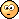?

Initialization is a phase in a variable's lifecycle within Swift. It consists of the following:

1. Declaration

2. Initialization

3. Utilization

4. Deallocation

Let's take look at some examples:

 State Syntax What's happening 1. Declaration `var a: Int` A variable gets declared; the type is defined and a space in memory is reserved; a name is assigned 2. Initialization `a = 10` A meaningful value is placed in the previously reserved space 3. Utilization `print(a)``a += 2` A value is taken from the memory and unitized (for example, when using print function), or it is modified (for example when using a subtraction operator). 4. Deallocation - Swift takes care of this for us!

Let's review the first 3 stages in detail:

#### Declaration

The declaration of a variable reserves space for it in the memory. At this time, the reserved space contains some information that's unrelated to our code - in other words - appears as garbage to us. Referring to our dresser analogy, this could be like a old sock and a random pair of pants that someone else has left behind.

How come there's still some data that belonged to some other code in the memory ?

When the computer deallocates or dismisses a variable, to save time, it only MARKS the memory as FREE or AVAILABLE, but it doesn't actually clean up previous data. In other words, it leaves the socks and pants in the drawers and empties them...hm.. later.

Now we can see why, if we tried to access a variable right after its declaration, it will result in an error - we would be trying to access some data that makes no sense!

#### Initialization

For this step we are assigning a meaningful initial value (hence the term Initialization) to our variable. Speaking of reserved drawers, we are now removing what was previously there and putting in what needs to be there.

After this step, we can safely utilize our variable. Let's add an initialization line to our original example:

``````var a: Int
a = 10
print(a)
``````

No more complaints in Playground!

The fun part is that in case we know the initial value we want our variable to store, we can perform 2 steps - declaration and initialization in one. We've already done it multiple times, so this variation is not unfamiliar to you:

``````var a: Int = 0 // or shorter version: var a = 10
print(a)
``````

Declaring an array is very similar. Here is an example of declaring an empty array:

``````var myArray: [Int]
myArray = []

// or in one line
var myArray: [Int] = []
``````

Here's an example of declaring an array with some elements:

``````var myArray: [Int]
myArray = [10, 12]

// or in one line
var myArray: [Int] = [10, 12]
``````

#### Initializing types

Another way to declare a variable and initialize it in one shot is to initialize its type and assign it to the variable we are declaring. The type initialization is done by adding parenthesis ( `()` ) after specifying the type. Here are a few examples:

``````// declaration AND initialization with default type values
var s = String() // ""
var i = Int() // 0
var f = Float() // 0.0
var d = Double() // 0.0
var b = Bool() // false
``````

And of course the arrays:

``````var a = [Int]()
``````

#### Utilization

We can use an array according to how we originally initialized it, or modify it as we see fit.

Like other variables, arrays can be constants (if we declare them using   `let`   keyword instead of  `var`  )

There are two ways to modify an array:

1. Assigning a completely new array to a previously declared variable:

``````var myVariableArray = [10, 12]
myVariableArray = [0, 2]

let myConstantArray = [10, 12]
myConstantArray = [0, 2] // Error
``````

This works exactly the same way as with other types of variables: we can reassign a value to a variable (first example) but not to a constant (second example).

2. Manipulating elements of an array. This is referred to as Mutability of an array.

Mutability is the ability to modify the value of a variable without reassigning a completely different value.

For example, we could change the first element of an array by assigning a new array to it with a different first element:

``````var myArray = [10, 12]
myArray = [11, 12]
``````

That, in theory, is perfectly valid. However, imagine we have an array of a hundred or, worse, a thousand elements?! If we begin adding and removing elements...just try to visualize the code. I will not be typing this example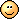So, mutability allows us to mutate an array without having to compose a brand new array for every desired mutation.

And what mutations can we perform on arrays?

Here are the most frequently used operations we can do with arrays:

• access each element by its index

• append a new element to the end

• insert a new element at a specific index

• remove an element at a specific index

#### Accessing an element

To access an element we can simply use square brackets and specify index of a desired element ( `[index]` ):

``````print(myArray) // 12
``````

Or we can replace an element at a given index:

``````myArray = 11
print(myArray) // 11
``````

#### Manipulating elements

Manipulating elements, such as adding or removing, requires using methods applicable to an array.

What's a method?

A method is a function.

What's a function?

A function is ...Hold your breath until the next part of this course. Using a method is very simple though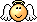.

So, we've got to use following most common methods of arrays:

• `append`   -  to add a new element at the end of an array. Here we need to provide a new element.

• `insert`  - to add a new element at a specific index. Here we need to provide a new element and the index at which we want a new value to be positioned.

• `remove`  - to remove (or delete) a existing element at a specific index. Here we need to provide the index of element we wish to remove.

And here's the syntax for the above:

``````var myArray = [10, 12]
myArray = [11, 12]

myArray.append(15) // [11, 12, 15]
myArray.insert(14, at: 2) // [11, 12, 14, 15]
myArray.remove(at: 1) // removed 12 => [11, 14, 15]
``````

And how does mutability apply to constants?

That one is simple: it doesn't apply at all! We cannot mutate an array that's a constant, only variable arrays are subject for mutation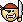!

Let's repeat:

• Arrays declared using keyword  `var`   are variable, can change (can mutate), or are simply mutable

• Arrays declared using keyword  `let`  are constant, cannot change (cannot mutate), or are simply immutable.

Yes, there are a couple more essential activities to perform with arrays!

#### Counting elements

There is a very important property to use with arrays,  `count` , which allows us to get the number of elements in an array:

``````var myArray = [10, 12]
print(myArray.count) // 2
``````

#### Browsing elements

Browsing (or walking) an array is another number one activity we'll be doing with arrays. And we already know what could be a suitable approach: for-in loops!

Here's an example to greet my friends. Test it in Playground:

``````var myFriends = ["Jenny", "Livia", "Paul"]
for myFriend in myFriends {
print("Hi, \(myFriend)!")
}
``````

And observe the result in the console:

```Hi, Jenny!
Hi, Livia!
Hi, Paul!```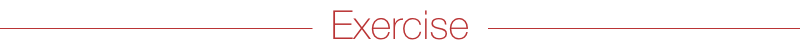Let's practice a bit. Say you played a game and your scores varied at each attempt:  122, 342, 107, 253, 471, 387. Calculate the average score and then add it as the first element in the original sequence!

``````// As usual, try to do it yourself!

// original scores
var scores = [122, 342, 107, 253, 471, 387]

// initial sum of scores
var sum = 0

// walk the array of scores
for score in scores {
sum += score
}

// calculate the average
let average = sum / scores.count //280

//insert the newly calulated average as the first element
scores.insert(average, at: 0)
``````

It depends on which game you played of course, but let's assume 280 is an excellent result!

Well, that's all good - lots of new knowledge. Let's apply it to our next challenge!

Remember, we have to calculate profits for 3 categories of sellable items at Jenny's cafe. Looks like it would be suitable to use an array with 3 elements of decimal numbers. Each element would represent a profit for each category in this particular order: coffee, dessert and food:

``````var profits = [0.0, 0.0, 0.0]
``````

Let's make a note of indexing here:

• 0 - coffee

• 1 - dessert

• 2 - food

Let's assume the initial values for our calculation period are all zeros (0.0: they must be decimal to account for cents).

And what's the logic behind calculating profit?

Oh, that! Jenny forgot to mention that previously. Here are the details for the last 2 months of operations (all numbers represent averages and include the cost of ingredients, the cost of the staff time to prepare and serve, and the sale price):

 Ingredient cost Service cost Price Portions sold per day on weekdays Portions sold per day on Saturday Coffee \$0.50 \$1.00 \$2.70 450 560 Dessert \$3.50 \$0 \$5.50 180 240 Food \$7.00 \$5.00 \$15 120 80

Oh, and she'd like to know total profit for all categories for the period of those 2 months!

What context should we remember from previous experiences?

Remember, the shop is closed on Sundays!

Start by calculating total profits for each category, taking in account the details above. Try doing it yourself first:

``````// Don't scroll just yet!

// initial profits for all categories
var profits = [0.0, 0.0, 0.0]

// profit calculation for 2 months
for day in 1 ... 60 {
if (day % 7 == 5) { // if Saturday
profits += (2.70 - (0.50 + 1.00)) * 450 // coffee
profits += (5.50 - (3.50 + 0.00)) * 180 // dessert
profits += (15.0 - (7.00 + 5.00)) * 120 // food
}
else if (day % 7 != 6) { // other days of the week excluding Sundays
profits += (2.70 - (0.50 + 1.00)) * 560 // coffee
profits += (5.50 - (3.50 + 0.00)) * 240 // dessert
profits += (15.0 - (7.00 + 5.00)) * 80 // food
}
}
``````

Does your code look similar? If not, it I hope it's better!

Next we need to figure which of the categories is the most profitable:

``````// Pause here, try it yourself!

// find out the most profitable category
if (profits > profits && profits > profits) { // compare the first element to the rest
print("Coffee is the most profitable category")
}
else if (profits > profits && profits > profits) { // compare the second element to the rest
print("Dessert is the most profitable category")
}
else if (profits > profits && profits > profits) { // compare the third element to the rest
print("Food is the most profitable category")
}
``````

Observe the result in console:

`Coffee is the most profitable category`

The final calculation is to find out the total profit for the given period:

``````// No cheating!

// initial total profit
var totalProfit = 0.0

// put together profits for all categories
for profit in profits {
totalProfit += profit
}

// preset the final result
print("The total profit for 2 months is \$\(totalProfit)")
``````

Observe the result in console:

`The total profit for 2 months is \$71328.0`

Let's put it all together:

``````// initial profits for all categories
var profits = [0.0, 0.0, 0.0]

// profit calculation for 2 months
for day in 1 ... 60 {
if (day % 7 == 5) { // if Saturday
profits += (2.70 - (0.50 + 1.00)) * 450 // coffee
profits += (5.50 - (3.50 + 0.00)) * 180 // dessert
profits += (15.0 - (7.00 + 5.00)) * 120 // food
}
else if (day % 7 != 6) { // other days of the week excluding Sundays
profits += (2.70 - (0.50 + 1.00)) * 560 // coffee
profits += (5.50 - (3.50 + 0.00)) * 240 // dessert
profits += (15.0 - (7.00 + 5.00)) * 80 // food
}
}

// find out the most profitable category
if (profits > profits && profits > profits) { // compare the first element to the rest
print("Coffee is the most profitable category")
}
else if (profits > profits && profits > profits) { // compare the second element to the rest
print("Dessert is the most profitable category")
}
else if (profits > profits && profits > profits) { // compare the third element to the rest
print("Food is the most profitable category")
}

// initial total profit
var totalProfit = 0.0

// put together profits for all categories
for profit in profits {
totalProfit += profit
}

// preset the final result
print("The total profit for 2 months is \$\(totalProfit)")
``````

And, observe the final result in console:

```Coffee is the most profitable category
The total profit for 2 months is \$71328.0```

Bonus challenge:  Take some time to optimize the code. Some tips:

• Use variables (or constants) instead of initial numbers, where suitable, and to store calculations that are performed multiple times.

• Optimize the calculations of the most profitable category; instead of rough approach, use a chain of if/else statements.

Wow, this was a challenging chapter, I must say! It will all settle in with practice!

• Swift offers three types of collections:

• Arrays

• Dictionaries

• Sets

• Array is a numerically ordered list

• All elements in an array must be of the same type. The type of the array's elements cannot change after it's been declared.

• Declaring elements' type can be done in 2 ways:

• Specify a type in brackets: `[Int]`.

• Let it be inferred by assigning elements.

• Initializing an empty array can be done in 2 ways:

• By assigning an empty array `[]`  if the type of elements is known

• By assigning a default value of an array initialization specifying the elements' type:  `[Int]()` .

• To access or modify the elements of an array, use the index, in brackets:

``````var colors = ["green", "yellow", "blue"]
colors = "red"
``````
• To add or remove items, use the following methods:

• `append`  to add an element at the end of an array

• `insert`  to add an element at an index

• `remove`  to remove an item at an index

• The `count`  property is used to get the number of elements in an array

• You can browse the elements of an array using a for-in loop

• The variables evolve according to a life cycle of 4 steps:

• Declaration: Creating a name of a variable and reserving space for it according to its type

• Initialization: Associating an initial value with a variable

• Utilization: Accessing or modifying the value

• Deallocation: Dismissing a variable (this is done 'automatically' and we don't have to worry about it in the code)

• A type can be initialized (producing a default value for the type) using the name of the type followed by parentheses: `Int()`